# JK Flip Flop | Diagram | Truth Table | Excitation Table

## Flip Flops-

Before you go through this article, make sure that you have gone through the previous article on Flip Flops.

We have discussed-

• A Flip Flop is a memory element that is capable of storing one bit of information.
• It is also called as Bistable Multivibrator since it has two stable states either 0 or 1.

There are following 4 basic types of flip flops-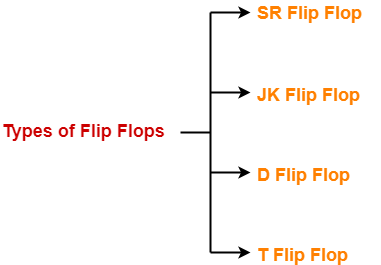1. SR Flip Flop
2. JK Flip Flop
3. D Flip Flop
4. T Flip Flop

## JK Flip Flop-

 JK flip flop is a refined & improved version of SR Flip Flop that has been introduced to solve the problem of indeterminate state that occurs in SR flip flop when both the inputs are 1.

In JK flip flop,

• Input J behaves like input S of SR flip flop which was meant to set the flip flop.
• Input K behaves like input R of SR flip flop which was meant to reset the flip flop.

## Construction of JK Flip Flop-

There are following two methods for constructing a JK flip flop-1. By using SR flip flop constructed from NOR latch
2. By using SR flip flop constructed from NAND latch

### 1. Construction of JK Flip Flop By Using SR Flip Flop Constructed From NOR Latch-

This method of constructing JK Flip Flop uses-

• SR Flip Flop constructed from NOR latch
• Two other connections

### Logic Circuit-

The logic circuit for JK Flip Flop constructed using SR Flip Flop constructed from NOR latch is as shown below-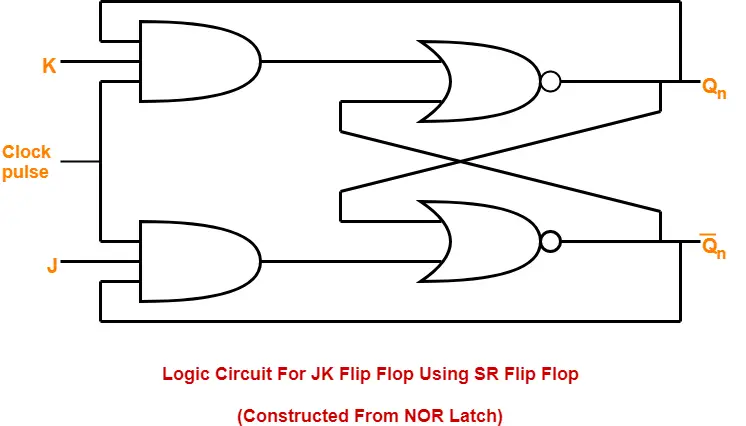### 2. Construction of JK Flip Flop By Using SR Flip Flop Constructed From NAND Latch-

This method of constructing JK Flip Flop uses-

• SR Flip Flop constructed from NAND latch
• Two other connections

### Logic Circuit-

The logic circuit for JK Flip Flop constructed using SR Flip Flop constructed from NAND latch is as shown below-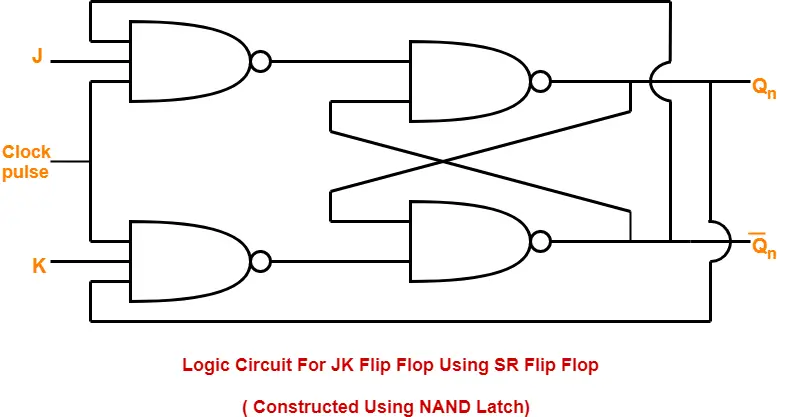### Logic Symbol-

The logic symbol for JK Flip Flop is as shown below-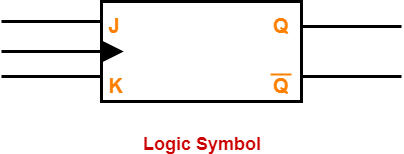### Truth Table-

The truth table for JK Flip Flop is as shown below-

 INPUTS OUTPUTS J K Qn (Present State) Qn+1 (Next State) 0 0 0 0 0 0 1 1 0 1 0 0 0 1 1 0 1 0 0 1 1 0 1 1 1 1 0 1 1 1 1 0

### Truth Table

The above truth table may be reduced as-

 INPUTS OUTPUTS REMARKS J K Qn (Present State) Qn+1 (Next State) States and Conditions 0 0 X Qn Hold State condition J = K = 0 0 1 X 0 Reset state condition J = 0 , K = 1 1 0 X 1 Set state condition J = 1 , K = 0 1 1 X Q’n Toggle state condition J = K = 1

### Characteristic Equation-

Draw a k map using the above truth table-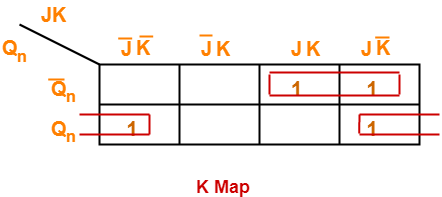From here-

Qn+1 = Q’n (JK + JK’) + Qn (J’K’ + JK’)

 Qn+1 = Q’nJ + QnK’

### Excitation Table-

The excitation table of any flip flop is drawn using its truth table.

 What is excitation table? For a given combination of present state Qn and next state Qn+1, excitation table tell the inputs required.

 Qn Qn+1 S R 0 0 0 X 0 1 1 X 1 0 X 1 1 1 X 0

## SR Flip Flop Vs JK Flip Flop-

Both JK flip flop and SR flip flop are functionally same.

The only difference between them is-

• In JK flip flop, indeterminate state does not occur.
• In JK flip flop, instead of indeterminate state, the present state toggles.
• In other words, the present state gets inverted when both the inputs are 1.

To gain better understanding about JK Flip Flop,

Watch this Video Lecture

Get more notes and other study material of Digital Design.

Watch video lectures by visiting our YouTube channel LearnVidFun.

SummaryArticle Name
JK Flip Flop | Diagram | Truth Table | Excitation Table
Description
JK flip flop is a refined and improved version of the SR flip flop. JK Flip Flop Construction, Logic Circuit Diagram, Logic Symbol, Truth Table, Characteristic Equation & Excitation Table are discussed.
Author
Publisher Name
Gate Vidyalay
Publisher Logo